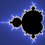# Problem 5b

Solve for $$x,y,z$$
$\frac { x }{ y } +\frac { y }{ z } +\frac { z }{ x } =\frac { y }{ x } +\frac { z }{ y } +\frac { x }{ z } =x+y+z=3$
This a part of my set NMTC 2nd Level (Junior) held in 2014.Note by Siddharth G
6 years, 6 months ago

This discussion board is a place to discuss our Daily Challenges and the math and science related to those challenges. Explanations are more than just a solution — they should explain the steps and thinking strategies that you used to obtain the solution. Comments should further the discussion of math and science.

When posting on Brilliant:

• Use the emojis to react to an explanation, whether you're congratulating a job well done , or just really confused .
• Ask specific questions about the challenge or the steps in somebody's explanation. Well-posed questions can add a lot to the discussion, but posting "I don't understand!" doesn't help anyone.
• Try to contribute something new to the discussion, whether it is an extension, generalization or other idea related to the challenge.
• Stay on topic — we're all here to learn more about math and science, not to hear about your favorite get-rich-quick scheme or current world events.

MarkdownAppears as
*italics* or _italics_ italics
**bold** or __bold__ bold
- bulleted- list
• bulleted
• list
1. numbered2. list
1. numbered
2. list
Note: you must add a full line of space before and after lists for them to show up correctly
paragraph 1paragraph 2

paragraph 1

paragraph 2

[example link](https://brilliant.org)example link
> This is a quote
This is a quote
    # I indented these lines
# 4 spaces, and now they show
# up as a code block.

print "hello world"
# I indented these lines
# 4 spaces, and now they show
# up as a code block.

print "hello world"
MathAppears as
Remember to wrap math in $$ ... $$ or $ ... $ to ensure proper formatting.
2 \times 3 $2 \times 3$
2^{34} $2^{34}$
a_{i-1} $a_{i-1}$
\frac{2}{3} $\frac{2}{3}$
\sqrt{2} $\sqrt{2}$
\sum_{i=1}^3 $\sum_{i=1}^3$
\sin \theta $\sin \theta$
\boxed{123} $\boxed{123}$

## Comments

Sort by:

Top Newest

$\dfrac{x}{y}+\dfrac{y}{z}+\dfrac{z}{x}\ge 3$ by AM-GM, with equality case if and only if $x=y=z$

Since $x+y+z=3$, then we know the only real solution is $(x,y,z)=\boxed{(1,1,1)}$

- 6 years, 6 months ago

Log in to reply

Good observation.

Note that the application of AM-GM assumes that the variables are positive.
Are there solutions over all reals?
What about over all complex numbers?

Staff - 6 years, 6 months ago

Log in to reply

$minimum\quad value\quad of\quad \frac { x }{ y } \quad +\quad \frac { y }{ z } +\frac { z }{ x } =3\quad \\ from\quad this\quad how\quad can\quad we\quad conclude\quad that\quad x=y=z????$

- 6 years, 6 months ago

Log in to reply

Hint: Make common denominator for$\frac{ x}{y} + \frac{y}{z} + \frac{z}{x} - \frac{ y}{x} - \frac{ z}{y} - \frac{ x}{z}$.
Factorize the numerator.

Staff - 6 years, 6 months ago

Log in to reply

$\frac{ x}{y} + \frac{y}{z} + \frac{z}{x} - \frac{ y}{x} - \frac{ z}{y} - \frac{ x}{z} = \frac{z-y}x+\frac{x-z}y+\frac{y-x}z=\frac{(z-y)zy+(x-z)xz+(y-x)yx}{xyz}=\frac{(y-z)(x-z)(y-x)}{xyz}=0$

Therefore $x,y,z\ne0$ and ($y=z$ or $x=z$ or $y=x$).

- 6 years, 6 months ago

Log in to reply

Great work.

This is a short step away from finishing the solution.

Staff - 6 years, 6 months ago

Log in to reply

When $y=z$, $\frac xy+1+\frac yx=\frac yx+1+\frac xy=x+2y=3$

$\frac xy+\frac yx=2$, $\frac xy=1$, $x=y$.

Therefore, $x=y=z$.

From $x+y+z=3$, we have $x=y=z=1$ as the only real solution.

- 6 years, 6 months ago

Log in to reply

x=1,y=1,z=1

- 6 years, 6 months ago

Log in to reply

Write a comment or ask a question... (1,1,1)

- 6 years, 6 months ago

Log in to reply

How do you know that there are no other solutions?

Staff - 6 years, 6 months ago

Log in to reply

x=y=z=1 therefore the answer is 3 ,basic algebra question

- 6 years, 6 months ago

Log in to reply

×

Problem Loading...

Note Loading...

Set Loading...